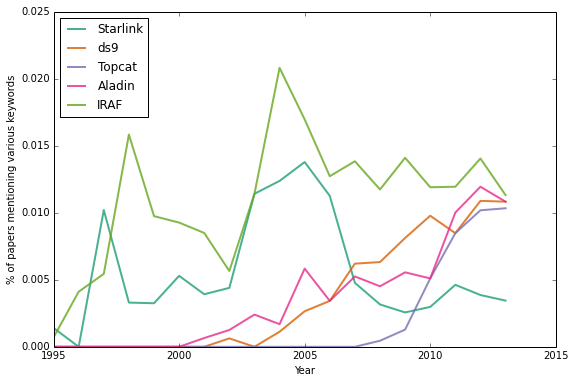Thomas P. Robitaille (Homepage)

NOTE: The background and results are discussed in this blog post.

Comments/improvements welcome! The source for this notebook lives on GitHub.This notebook was created using Python 3.3.

Introduction¶

In this notebook, I will show how we can use the SAO/NASA ADS developer API to access statistics about how often words/phrases are used in acknowledgment sections of papers. To run the notebook, you will need an ADS API developer key set in the ADS_DEV_KEY environment variable. See the adsabs-dev-api repository for more details on obtaining a key.

As an aside, we will make use of the brewer2mpl package in order to improve the look of the plots:

In :
import brewer2mpl
mpl.rcParams['axes.color_cycle'] = brewer2mpl.get_map('Dark2', 'qualitative', 7).mpl_colors
mpl.rcParams['figure.figsize'] = (9,6)

Querying the database¶

We start off by getting the developer key from the environment variable and setting the base URL for the query:

In :
import os

Next, we can start preparing an example query, which is stored as a dictionary of keyword/value pairs:

In :
params = {}

The q parameter is used to specify the query. In our case we want to query the acknowledgment section of papers, so we use the ack:<string> syntax:

In :

We then specify which fields we want the query to return. For the purposes of this notebook, we only care about the publication date:

In :
params['fl'] = 'pubdate'

Finally, we set the maximum number of rows for each query and the API key:

In :
params['rows'] = '10'  # use a small value for now
params['dev_key'] = DEV_KEY

Since the results returned are split up, with a limit of 200 results for each request, we have to make the query multiple times, specifying a different starting point (which we give with the start parameter) every time. Let's start off by executing the request for start==0:

In :
import requests
params['start'] = 0
r = requests.get(BASE_URL, params=params)

We can then parse the results (which are returned as a JSON object):

In :
import simplejson

Let's now access the results by iterating over data['results']['docs']. Each result element is a dictionary containing the requested fields and a few ohter default fields:

In :
data['results']['docs']
Out:
{'bibcode': '2013MNRAS.434.3423G', 'id': '9873361', 'pubdate': '2013-10-00'}

so we can extract all the publication dates with:

In :
for d in data['results']['docs']:
print(d['pubdate'])
2013-10-00
2013-10-00
2013-10-00
2013-10-00
2013-10-00
2013-10-00
2013-10-00
2013-10-00
2013-10-00
2013-10-00

These are in the YYYY-MM-DD format, but note that the day is zero in the above cases (which the API docs say is expected behavior). For the purposes of plotting these dates, we only care about the year:

In :
year = d['pubdate'].split('-')
print(year)
2013

In the remainder of this notebook, we will care only about yearly statistics (due to small number statistics) but one could also repeat the same analysis on a monthly basis.

A more complete querying function¶

We can now put all of the above together into a single function that returns the publication years for a given string:

In :
import os

def query_acknowledgments(word):

# Set query parameters
params = {
'q': 'ack:{0:s},property:REFEREED'.format(word),
'fl': 'pubdate',
'rows': '200',
'dev_key': DEV_KEY
}

import requests
params['start'] = 0

processed = 0

pub_years = []
while True:

# Execute the query
r = requests.get(BASE_URL, params=params)

# Check if anything went wrong
if r.status_code != requests.codes.ok:
sys.stderr.write("error retrieving results: {0:s}\n".format(e['error']))
continue

# Extract results
import simplejson
for d in data['results']['docs']:
pub_years.append(float(d['pubdate'].split('-')))

# Update starting point
params['start'] += data['meta']['count']

# Check if finished
if params['start'] >= data['meta']['hits']:
break

import numpy as np
return np.array(pub_years)

We can now test out this function:

In :
pub_years
Out:
array([ 2013.,  2013.,  2013., ...,  1995.,  1995.,  1995.])

Plotting the results¶

We first set the years that we are going to make plots for:

In :
YEARS = list(range(1995, 2014))
YEARS
Out:
[1995,
1996,
1997,
1998,
1999,
2000,
2001,
2002,
2003,
2004,
2005,
2006,
2007,
2008,
2009,
2010,
2011,
2012,
2013]

Let's now count the results from above for each year:

In :
query_count = np.array([np.sum(pub_years == year) for year in YEARS])
In :
plt.plot(YEARS, query_count)
_ = plt.xlabel("Year")
_ = plt.ylabel("Number of papers mentioning SIMBAD")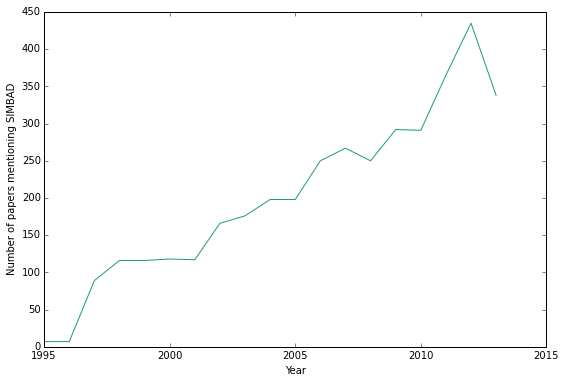Normalizing by the number of papers¶

Let's try and find out how many papers were published each year. We don't want to retrieve all bibcodes ever since this would be slow, but we can make use of the fact that a query returns a hits parameter giving the total number of results, then search on a year by year basis.

In :
def total_number(year):

params = {
'q': 'pubdate:{0:s},property:REFEREED'.format(year),
'dev_key':DEV_KEY,
'rows':1
}

import requests
r = requests.get(BASE_URL, params=params)

import simplejson

return data['meta']['hits']
In :
total_number('2012')
Out:
284484

Let's now query once and for all the number of papers for every year in the range we are interested in:

In :
TOTAL_COUNT = []

for year in YEARS:
date = '{0:04d}'.format(year)
TOTAL_COUNT.append(total_number(date))

TOTAL_COUNT = np.array(TOTAL_COUNT)
In :
plt.plot(YEARS, TOTAL_COUNT)
plt.ylim(0, 400000)
_ = plt.xlabel("Year")
_ = plt.ylabel("Total number of papers")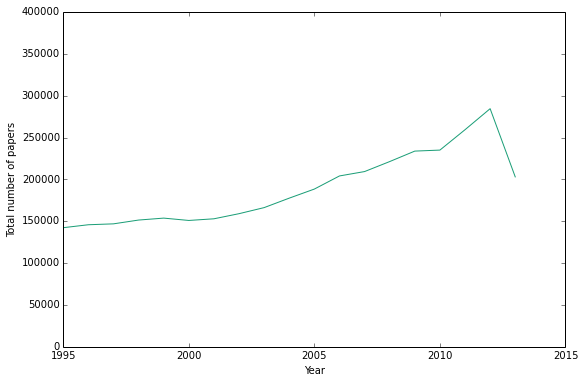Let's now apply this to the query for 'simbad' that we made previously:

In :
plt.plot(YEARS, query_count / TOTAL_COUNT * 100.)
plt.xlabel("Year")
Out:
<matplotlib.text.Text at 0x1046f1610>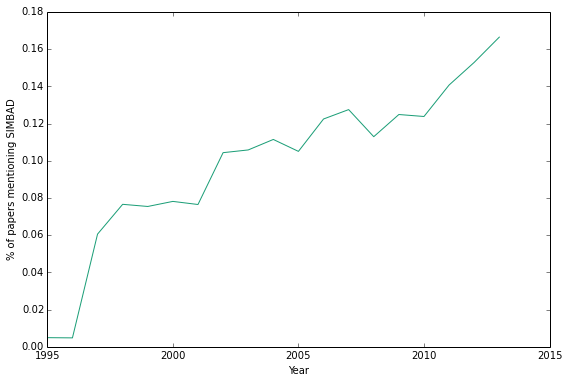Let's finally wrap up the plotting code into a single function to make it easier to overplot different keywords:

In :
def plot_yearly_trend(keyword, label=None):
pub_years = query_acknowledgments(keyword)
query_count = np.array([np.sum(pub_years == year) for year in YEARS])
plt.plot(YEARS, query_count / TOTAL_COUNT * 100., label=label, lw=2, alpha=0.8)
In :
plt.legend(loc=2)
_ = plt.xlabel("Year")
_ = plt.ylabel("% of papers mentioning various keywords")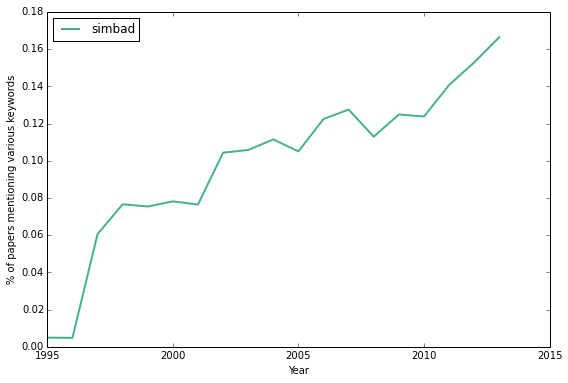Examples¶

The following is quite slow because this acknowledgment is reasonably popular:

In :
plot_yearly_trend('Astrophysics Data System', label='Astrophysics Data System')
plt.legend(loc=2)
_ = plt.xlabel("Year")
_ = plt.ylabel("% of papers mentioning various keywords")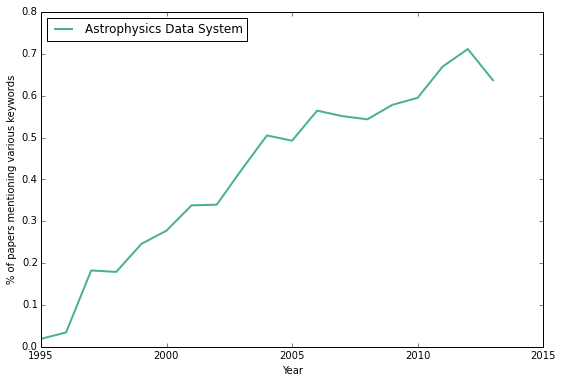Online databases¶

The following is quite slow because all these keywords are reasonably popular

In :
plot_yearly_trend('vizier', label='Vizier')
plot_yearly_trend('ned', label='NED')
plt.legend(loc=2)
_ = plt.xlabel("Year")
_ = plt.ylabel("% of papers mentioning various keywords")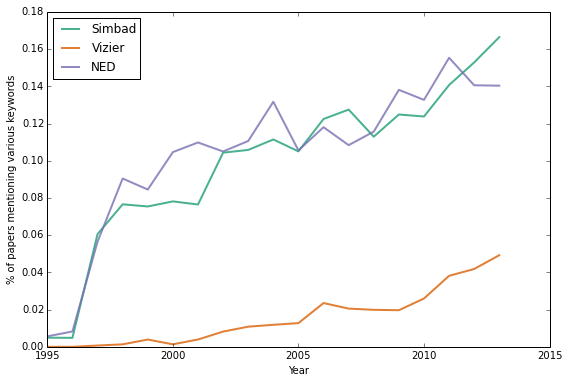Programming languages¶

In :
plot_yearly_trend('idl', label='IDL')
plot_yearly_trend('python', label='Python')
plot_yearly_trend('fortran', label='Fortran')
plot_yearly_trend('perl', label='perl')
plt.legend(loc=2)
_ = plt.xlabel("Year")
_ = plt.ylabel("% of papers mentioning various keywords")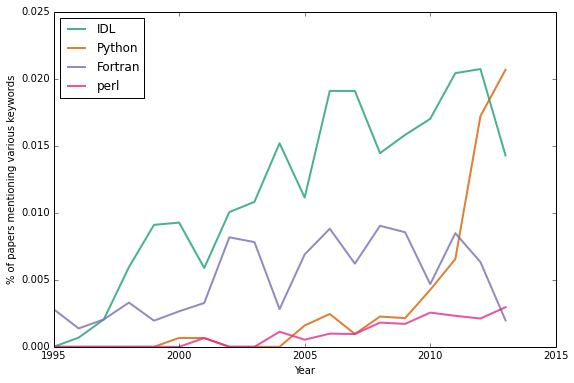Tools¶

In :